# Category PLUMBER’S AND. PIPE FITTER’S. CALCULATIONS MANUAL

## HOW MANY GALLONS?

How many gallons does that tank hold? Do you know how to determine the capacity of a tank? Well if you don’t, you’re about to see an easy way to find out. Before you can start to do your math, you have to know if you will be working with measurements in inches or in feet. You also have to know that the tank diameter is known as D and the tank height is H. We are looking for the tank capacity in gallons, which we will identify in our formula with the letter G.

When the measurements for a tank are expressed in inches, you will use a factor of 0.0034 in your formula. Tanks that are measured in terms of feet require a factor of 7.5. For our example, we are going to measure our tank in inches. This particular tank is 18 inches in diameter and 60 inches in height. The generic formula for this type of problem is as follows: G = d2 X h X 0.0034. We know some of the variables, so we have to put them into our equation.

The diameter of our tank is 18 inches and the height is 60 inches, so our formula will look like this: G = 182 X 60 X 0.0034. What is 182? It’s 324. This is found by multiplying 18 by itself or 18 X 18. Now we know that we are going to multiply 324 by 60 as we follow our formula. This will give us a number of 19440. The last step of our formula is to multiply 19440 by the 0.0034 factor. This will result in an answer of 66.10. We are looking for the maximum capacity of the tank, so we adjust the 66.10 to an even 66 gallons. That wasn’t too bad, was it?

CYLINDER-SHAPED CONTAINERS

cylinder-shaped containers could be tanks, pipes, or any other number of de­vices. What happens if you want to know the holding capacity of such an ob­ject? You are going to need to use a formula that involves the radius (R) of the object, the diameter of the object (D), the height (H) of the object, and the value assigned to л, which is 3.1416. Our goal is to find the volume (V) ca­pacity of a cylinder. There are two types of formulas that can be used to de­termine the capacity of a cylinder, so let’s take them one at a time.

The first formula that we are going to use looks like this: V = л r2 h. An­other way to find the answer is to have V = л divided by 4 d2 h. Either for­mula will give you the same answer, it’s just a matter of choosing one formula over another, based on your known elements of the question.

## PIPING MATH

This section will profile formulas that can help you when working with pipes. Rather than talk about them, let’s look at them.

What plumber hasn’t had to figure the grading for a drainage pipe? De­termining the amount of fall needed for a drainpipe over a specified distance is no big mystery. Yet, I’ve known good plumbers who had trouble with cal­culating the grade of their pipes. In fact, some of them were so unsure of themselves that they started at the end of their runs and worked backwards, to the beginning, to insure enough grade. Not only is this more difficult and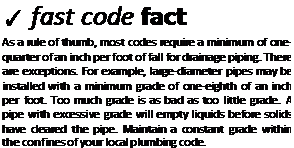time consuming, there is still no guar­antee that there will be enough room for the grade. Knowing how to figure the grade, fall, pitch, or whatever you want to call it, for a pipe is essential in the plumbing trade. And, it’s not diffi­cult. Let me show you what I mean.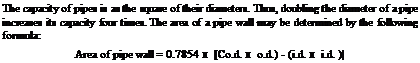In a simple way of putting it, as­sume that you are installing a pipe that is 20 feet long and that will have a grade of V4-inch per foot. What will the drop from the top of the pipe be from one end to the other? At a grade of V-t-inch per foot, the pipe will drop one inch for every four feet it travels. A 20-foot piece of pipe will require a 5- inch drop in the scenario described. By dividing 4 into 20, I got an answer of 5, which is the number of inches of drop. That’s my simple way of doing it,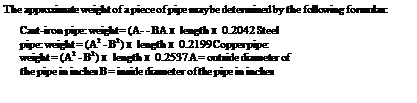FIGURE 1.6 ■ Piping. (Courtesy of McGraw-Hill)

FIGURE 1.7 ■ Determining pipe weight. (Courtesy of McGraw-Hill)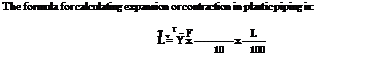FIGURE 1.8 ■ Expansion in plastic piping. (Courtesy of McGraw-Hill)

 The formulas for pipe radiation of heat are as follows: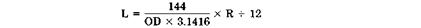D = outside diameter (OD) of pipe L = length of pipe needed in feet R = square feet of radiation needed

FIGURE 1.9 ■ Formulas for pipe radiation of heat. (Courtesy of McGraw-Hill)

but now let me give you the more proper way of doing it with a more so­phisticated formula.

If you are going to use the math formula, you must know the terms asso­ciated with it. Run is the horizontal distance that the pipe you are working with will cover, and this measurement is shown as the letter R. Grade is the slope of the pipe and is figured in inches per foot. To define grade in a for­mula, the letter G is used. Drop is the amount down from level or in more plumber-friendly words, it’s the difference in height from one end of the pipe to the other. As you might guess, drop is known by the letter D. Now let’s put this into a formula. To determine grade with the formula above, you would be looking at something like this: D = G X R. If you know some of the vari­ables, you can find the rest. For example, if you know how far the pipe has to run and what the maximum amount of drop can be, you can determine the grade. When you know the grade and the length of the run, you can deter­mine the drop. I already showed you how to find the drop if you know grade and run numbers. So, let’s assume an example where you know that the drop is 15 inches and the run is 60 feet, what is the grade? To find the answer, you divide the drop by the run, in this case you are dividing 15 by 60. The answer is.25 or V4-inch per foot of grade.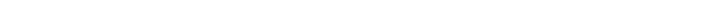Let me give you a few illustrations here that will help you deal with tempera­tures, heat loss, and mixing temperatures.

 Temperature may be expressed according to the Fahrenheit (F) scale or the Celsius (Cl scale. To convert rC to °F or F to C, use the following formulas: F = 1.8 x C * 32 C = 0.55555555 x ‘F – 32 ■C = F – 32 1.8 F = ( . x 1.8 + 32

 FIGURE 1.10 ■ Temperature conversion. (Courtesy of McGraw-Hill)

 To figure the Anal temperature when two different temperatures of water are mixed together, use the following formula: (A x C) s – (B x D) A В A = weight of lower temperature water В = weight of higher temperature water C = lower temperature D = higher temperature

 FIGURE 1.11 ■ Computing water temperature. (Courtesy of McGraw-Hill)

 Radiation 3 ft of 1-in. pipe equal 1 ft" R. 2’Li lineal ft of 1’/ i-in. pipe equal 1 ft" R. Hot water radiation gives off 150 Btu/ft- R/hr. Steam radiation gives off 240 Btu/ft – R/hr. On greenhouse heating, figure ~/л ft ‘ R/fC glass. 1 ft“ of direct radiation condenses 0.25 lb water/hr.

 -100°-30° QC Base temperature -73 -100 -148 -68 -90 -130 -62 -80 -112 -57 -70 -94 -51 -60 -76 -46 -50 -58 -40 -40 -40 -34.4 -30 -22 -28.9 -20 -4 -23.3 -10 14 -17.8 0 32 -17.2 1 33.8 -16.7 2 35.6 -16.1 3 37.4 -15.6 4 39.2 -15.0 5 41.0 -14.4 6 42.8 -13.9 7 44.6 -13.3 8 46.4 -12.8 9 48.2 -12.2 10 50.0 -11.7 11 51.8 -11.1 12 53.6 -10.6 13 55.4 -10.0 14 57.2 ЗГ-7Г °С Base temperature °F -0.6 31 87.8 0 32 89.6 0.6 33 91.4 1.1 34 93.2 1.7 35 95.0 2.2 36 96.8 2.8 37 98.6 3.3 38 100.4 3.9 39 102.2 4.4 40 104.0 5.0 41 105.8 5.6 42 107.6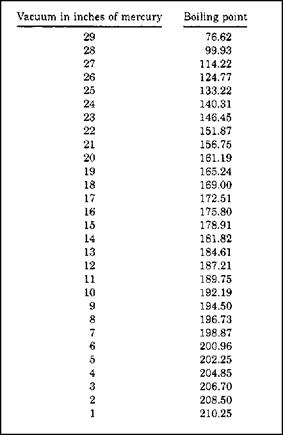FIGURE 1.14 ■ Boiling points of water based on pressure. (Courtesy of McGraw-Hill)

## GENERAL TRADE MATHEMATICS

 T

his book is your ticket to smooth sailing when it comes to doing the math for plumbing and pipe fitting. Most of the work is already done for you when you consult the many tables and references contained in these pages. Why waste time with calculators and complicated mathematical equations when you can turn to the ready-reference tables here and have the answers at your fingertips? There is no reason to take the difficult path when you can put your field skills to better use and make more money.

A few words of advice are needed here. Our country uses multiple plumb­ing codes. Every code jurisdiction can adopt a particular code and amend it to their local needs. It is impossible to provide one code source to serve every plumber’s needs. The code tables in this book are meant to be used as repre­sentative samples of how to arrive at your local requirements, but they are not a substitution for your regional code book. Always consult your local code be­fore installing plumbing.

The major codes at this time are the International Plumbing Code and the Uniform Plumbing Code. Both are excellent codes. There have been many code developments in recent years. In addition to these two major codes, there are smaller codes in place that are still active. I want to stress that this is not a handbook to the plumbing code,- this is a calculations manual. If you are interested in a pure code interpretation, you can review one of my other Mc­Graw-Hill books entitled: International and Uniform Plumbing Codes Handbook.

 A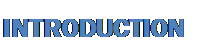re you a plumber or pipe fitter who dislikes doing the math that is re­quired in your trade? If so, this book may be one of the best tools that you can put in your truck or office. Why? Because it does much of the math calculations for you. That’s right, the tables and visual graphics between these pages can make your life much easier and more profitable.

R. Dodge Woodson, the author, is a 30-year veteran of the trade who has been in business for himself since 1979. He knows what it takes to win in all financial climates as both a business owner and tradesman. This is your chance to learn from an experienced master plumber and, what is even better, you don’t have to study and memorize formulas. All you have to do is turn to the section of this professional reference guide that affects your work and see the answers to your questions in black and white. How much easier could it be?

Mathematical matters are not the only treasures to be found here. You will find advice on how to comply with the plumbing code quickly, easily, and without as much thought on your part.

The backbone of this book is math for the trades, but there is much more. There is a section on troubleshooting that is sure to save you time, frustration, and money. Find out what you may need to know about septic systems. In ad­dition to phase-specific math solutions, there is an appendix that is full of ref­erence and conversion tables for day-to-day work situations.

Take a moment to scan the table of contents. You will see that the pres­entation of material here is compiled in logical, accessible, easy-to-use chap­ters. Flip through the pages and notice the tip boxes and visual nature of the information offered. You don’t have to read much, but you will find answers to your questions.

If you are looking for a fast, easy, profitable way to avoid the dense read­ing and complicated math that is needed in your trade, you have found it. Once you put this ready reference guide at your fingertips, you will be able to concentrate on what you do best without the obstacles that may steal your time and your patience. Packed with 30 years of experience, you can’t go wrong by using Woodson’s resources to make you a better tradesman.

ix

This page intentionally left blank.

 M

ath is not always a welcome topic among tradespeople. As much as math may be disliked, it plays a vital role in the trades, and plumb­ing and pipe fitting are no exceptions. In fact, the math requirements for some plumbing situations can be quite complicated. When people think of plumbers, few thoughts of scholarly types come to mind. I expect that most people would have trouble envisioning a plumber sitting at a drafting table and performing a variety of mathematical functions involving geometry, al­gebra, and related math skills. Yet, plumbers do use high-tech math in their trade, sometimes without realizing what they are doing.Think about your last week at work. Did you work with degrees of an­gles? Of course you did. Every pipe fitting you installed was an example of angles. Did you grade your drainage pipe? Sure you did, and you used frac­tions to do it. The chances are good that you did a lot more math than you realized. But, can you find the volume of a water heater if the tank is not marked for capacity? How much water would it take to fill up a 4-inch pipe that is 100 feet long? You might need to know if you are hauling the water in for an inspection test of the pipe. How much math you use on a daily basis is hard to predict. Much of the answer would depend on the type of work you do within the trade. But, it’s safe to say that you do use math on a daily basis.

I’ve taught a number of classes for plumbers and plumbing apprentices. Math is usually the least appreciated part of those classes. Experience has showed me that stu­dents resist the idea of learning math skills.

I remember when I took academic levels of math in school and thought that I’d never use it. Little did I know back then how valuable the skills I was learning would be.

1

 A or a Area, acre AWG American Wire Gauge В or b Breadth bbl Barrels bhp Brake horsepower BM Board measure Btu British thermal units BWG Birmingham Wire Gauge В & S Brown and Sharpe Wire Gauge (American Wire Gauge C of g Center of gravity cond Condensing cu Cubic cyl Cylinder D or d Depth, diameter dr Dram evap Evaporation F Coefficient of friction; Fahrenheit F or f Force, factor of safety ft (or ‘I Foot ft lb Foot pound fur Furlong gal Gallon gi Gill ha Hectare H or h Height, head of water HP horsepower IHP Indicated horsepower in for ") Inch L or l Length lb Pound lb/sq in. Pounds per square inch mi Mile o. d. Outside diameter (pipes) oz Ounces pt Pint P or p Pressure, load psi Pounds per square inch R or r Radius rpm Revolutions per minute sq ft Square foot sq in. Square inch sq yd Square yard T or t Thickness, temperature temp Temperature V or v Velocity vol Volume W or w Weight W. I. Wrought iron

FIGURE 1.1 ■ Abbreviations. (Courtesy of McGraw-Hill)

While I’m not a rocket scientist, I can take care of myself when it comes to do­ing math for trade applications.

I assume that your time is valuable and that you are not interested in a col­lege course in mathematics by the end of this chapter. We’re on the same page of the playbook. I’m going to give you concise directions for solving mathematical problems that are related to plumbing and pipefitting. We won’t be doing an in-depth study of the history of numbers, or anything like that. The work we do here will not be too difficult, but it will prepare you for the hurdles that you may have to clear as a thinking plumber. So, let’s do it.

The quicker we start, the quicker we can finish.

BENCHMARKS

Before we get into formulas and exercises, we need to establish some bench­marks for what we will be doing. It always helps to understand the termi­nology being used in any given situation, so refer to Figure 1.1 for reference to words and terms being used as we move forward in this chapter. The in­formation in Figure 1.2 shows you some basic formulas that can be appliedFIGURE 1.2 ■ Useful formulas. (Courtesy of McGraw-Hill)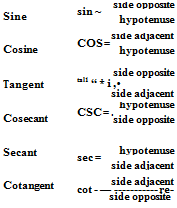FIGURE 1.3 ■ Trigonometry. (Courtesy of McGraw-Hill)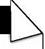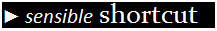to many mathematical situations. Trigonometry is a form of math that can send some people in the opposite direc­tion. Don’t run, it’s not that bad. Figure 1.3 provides you with some basics for trigonometry, and Figure 1.4 describes the names of shapes that contain a vari­ety of sides. Some more useful formulas are provided for you in Figure 1.5. Just in what I’ve provided here, you are in a much better position to solve mathe­matical problems. But, you probably want, or need, a little more explana­tion of how to use your newfound resources. Well, let’s do some math and see what happens.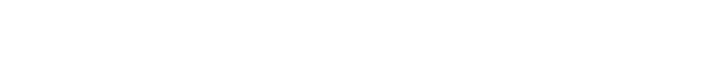Pentagon 5 sides Hexagon 6 sides Heptagon 7 sides Octagon 8 sides Nonagon 9 sides Decagon 10 sides

FIGURE 1.4 ■ Polygons. (Courtesy of McGraw-Hill)

 Parallelogram Pyramid Rectangle Rectangular prism Sphere Square Triangle Trapezoid Cone

 Area = base x distance between the two parallel sides Area = ‘h perimeter of base x slant height + area of base Volume = area of base x l/> of the altitude Area = length X width Volume = width x height x length Area of surface = diameter x diameter X 3.1416 Side of inscribed cube = radius x 1.547 Volume = diameter x diameter x diameter X 0.5236 Area = length x width Area = one-half of height times base Area = one-half of the sum of the parallel sides x the height Area of surface = one-half of circumference of base X slant height + area of base Volume = diameter x diameter x 0.7854 x one-third of the altitude

 Cube Ellipse Cylinder

 Volume = width X height X length Area = short diameter x long diameter x 0.7854 Area of surface = diameter x 3.1416 X length + area of the two bases Area of base = diameter x diameter x 0.7854 Area of base = volume – e length Length = volume – e area of base Volume = length x area of base Capacity in gallons = volume in inches + 231 Capacity of gallons = diameter x diameter x length x 0.0034 Capacity in gallons = volume in feet x 7.48 Circumference = diameter X 3.1416 Circumference = radius x 6.2832 Diameter = radius x 2 Diameter = square root of = (area – e 0.7854) Diameter = square root of area x 1,1233

 Circle